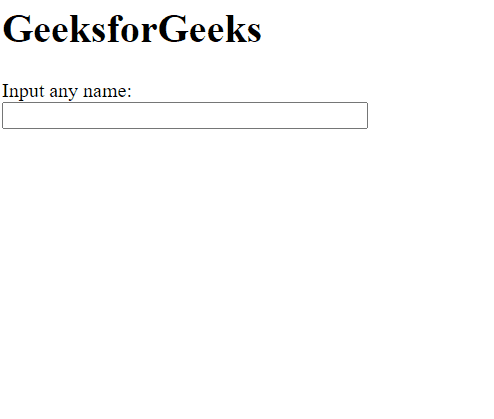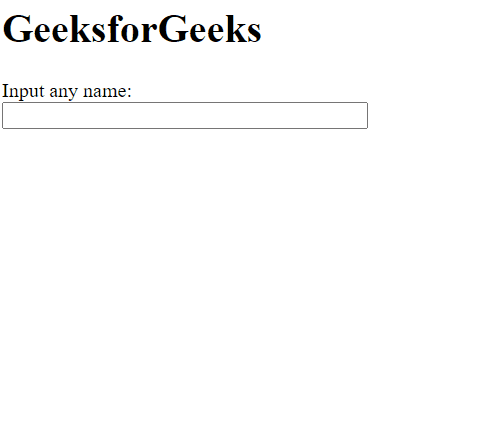Related Articles
script.aculo.us Autocompleter.Local choices Option
• Last Updated : 20 Nov, 2020

The script.aculo.us library is a cross-browser library that aims at improving the user interface of a website. The Autocompleter can be used for providing auto-completion support for text fields in the webpage. The local Autocompleter is used when auto-completion options are given as an array to the Autocompleter method to display as the choices.

The Autocompleter.Local choices option is used to define the number of auto-completion choices to be offered to the user.

Syntax:

```{ choices: number }
```

Values:

• number: This option takes a number which indicates a number of choices to offer. The default value is 10.

Example 1: In this example, we have set the number of choices to 5, therefore 5 choices are offered.

## HTML

 ```<``html``>`` ` `<``head``>``    ````    ``<``script` `type``=``"text/javascript"` `        ``src``=``"prototype.js"``>``    ```` ` `    ``<``script` `type``=``"text/javascript"` `src``=``"scriptaculous.js?load = effects,controls"``>``    `````` ` `<``body``>``    ``<``h1``>GeeksforGeeks`` ` `    ``<``label` `for``=``"GeeksforGeeks"``>``        ``Input any name:``    ````     ` `    ``<``br` `/>``    ``<``input` `id``=``"GeeksforGeeks"` `autocomplete``=``"off"` `        ``size``=``"40"` `type``=``"text"` `value``=``""` `/>`` ` ` ` `    ``<``div` `class``=``"autocomplete"` `id``=``"names"` `        ``style``=``"display:none"``>``    ```` ` `    ``<``script` `type``=``"text/javascript"``>`` ` `        ``// Array to be used as choices``        ``var names = [``            ``'Ab',``            ``'Abc',``            ``'Abcd',``            ``'Abcde',``            ``'Abcdef',``            ``'Abcdefg'``        ``];`` ` `        ``// Initialize the Autocompleter``        ``new Autocompleter.Local('GeeksforGeeks',``            ``'names', names, {`` ` `            ``// Specify the number of choices``            ``// to be displayed``            ``choices: 5``        ``});``    `````` ` ``

Output:Example 2: In this example, we have set the number of choices to 7, therefore 7 choices are offered.

## HTML

 ```<``html``>`` ` `<``head``>``    ````    ``<``script` `type``=``"text/javascript"` `        ``src``=``"prototype.js"``>``    ```` ` `    ``<``script` `type``=``"text/javascript"` `src``=``"scriptaculous.js?load = effects,controls"``>``    `````` ` `<``body``>``    ``<``h1``>GeeksforGeeks`` ` `    ``<``label` `for``=``"GeeksforGeeks"``>``        ``Input any name:``    ````     ` `    ``<``br` `/>``    ``<``input` `id``=``"GeeksforGeeks"` `autocomplete``=``"off"``        ``size``=``"40"` `type``=``"text"` `value``=``""` `/>`` ` `    ``<``div` `class``=``"autocomplete"` `id``=``"names"` `        ``style``=``"display:none"``>``    ```` ` `    ``<``script` `type``=``"text/javascript"``>`` ` `        ``// Array to be used as choices``        ``var names = [``            ``'Ab',``            ``'Abc',``            ``'Abcd',``            ``'Abcde',``            ``'Abcdef',``            ``'Abcdefg'``        ``];`` ` `        ``// Initialize the Autocompleter``        ``new Autocompleter.Local('GeeksforGeeks',``            ``'names', names, {`` ` `            ``// Specify the number of choices``            ``// to be displayed``            ``choices: 7``        ``});``    `````` ` ``

Output:My Personal Notes arrow_drop_up